# Diy servo driver (microcontroller)

Thanks to help of Ray Edgley I publish the 14A continuous (30A peak) analog and digital motor driver. Project is designed mostly for myrobotlab users but it may be used by other peoples. Driver can not be produced and selled

This is not the final version but is fully working with AM and SSM mode.

I think the PCB is the final version, its fully fit to VNH2SP30 shield.

One driver can controll 2 motors 14A each, max Voltage is 16V!.

This Driver have 2 mode of control, AM (analog mode) and SSM (servo signal mode) , in plans is third mode by I2C.

In analog mode AM driver control the motor by 0-5V signal to REF inputs, the POT inputs are for measure the motor position and set it to REF position.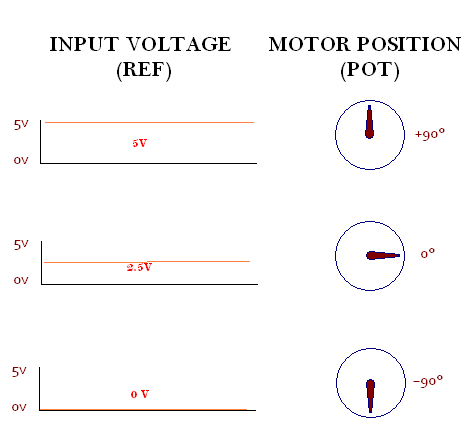In servo signal mode SSM driver control the motor by the input servo signal from 0.9ms to 2.4ms in 20ms loop connected to REF input. Motor position is controled by POT input like in analog mode.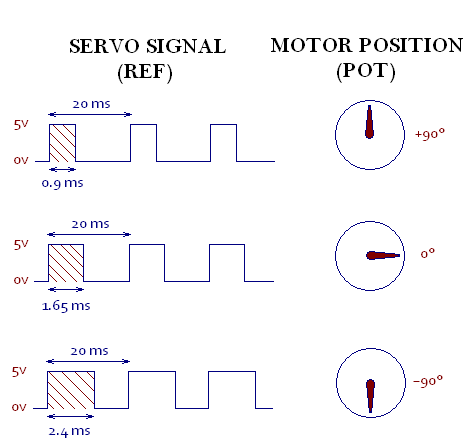To chose the mode simply put the jumper in control pins. If the jumper is with +5V the control will be SSM, if the jumper is to gnd then will be AM. The third option will be with no jumper.

Driver has many option like END SWITCH secure, if one of the POT input is connected GND the driver stops on actually position, this work in both of mode.
In SSM mode you may activate/deactivate driver output simply by attach or detach in myrobotlab or unpin POT connection.

Thanks to RAY Edgley Driver have PID regulation thanks to this driver may be set as need, but be carefull changing settings this may results in the driver not being able to control it.

Driver have also Deadband control, this alows the control of positioning accuracy.

CENNECTIONS EXAMPLE: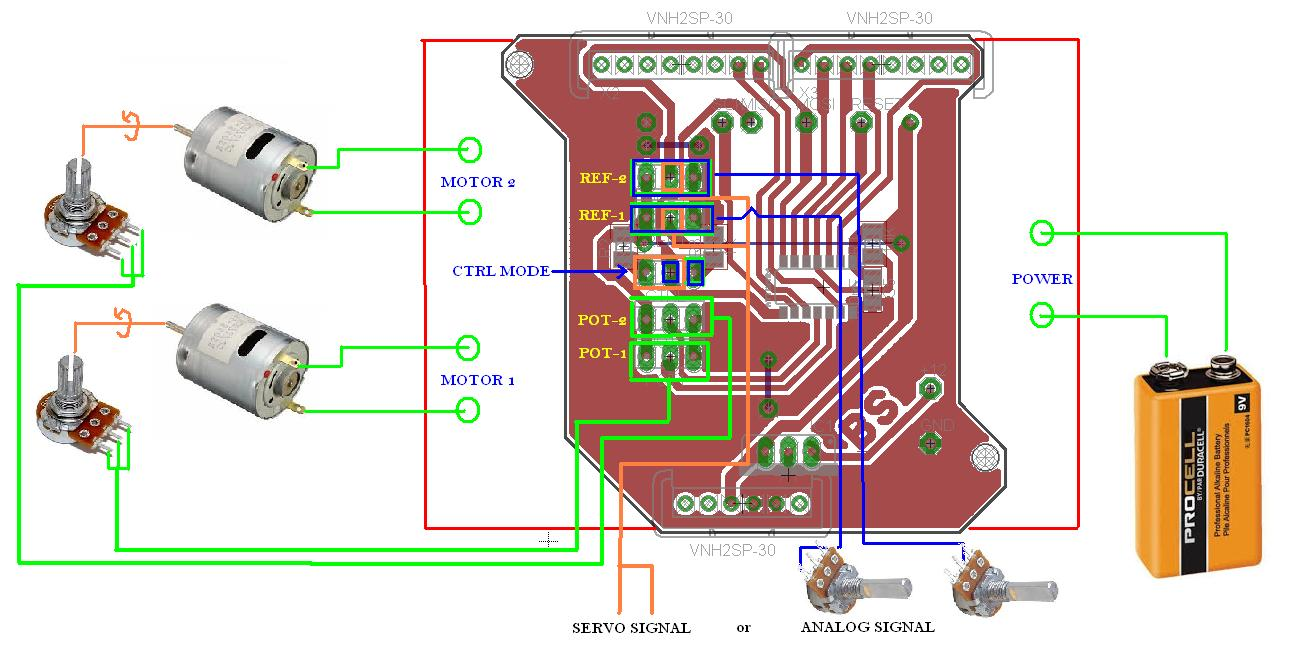Files is in free Cadsoft Eagle

List of parts:

- Attiny44 or Attiny84

- 7805 Voltage regulator

- 2 x 100nF capacitor

- 10K resistor

-  2 x 1K resistor

and... VNH2SP30 dual shield for 2 motors controll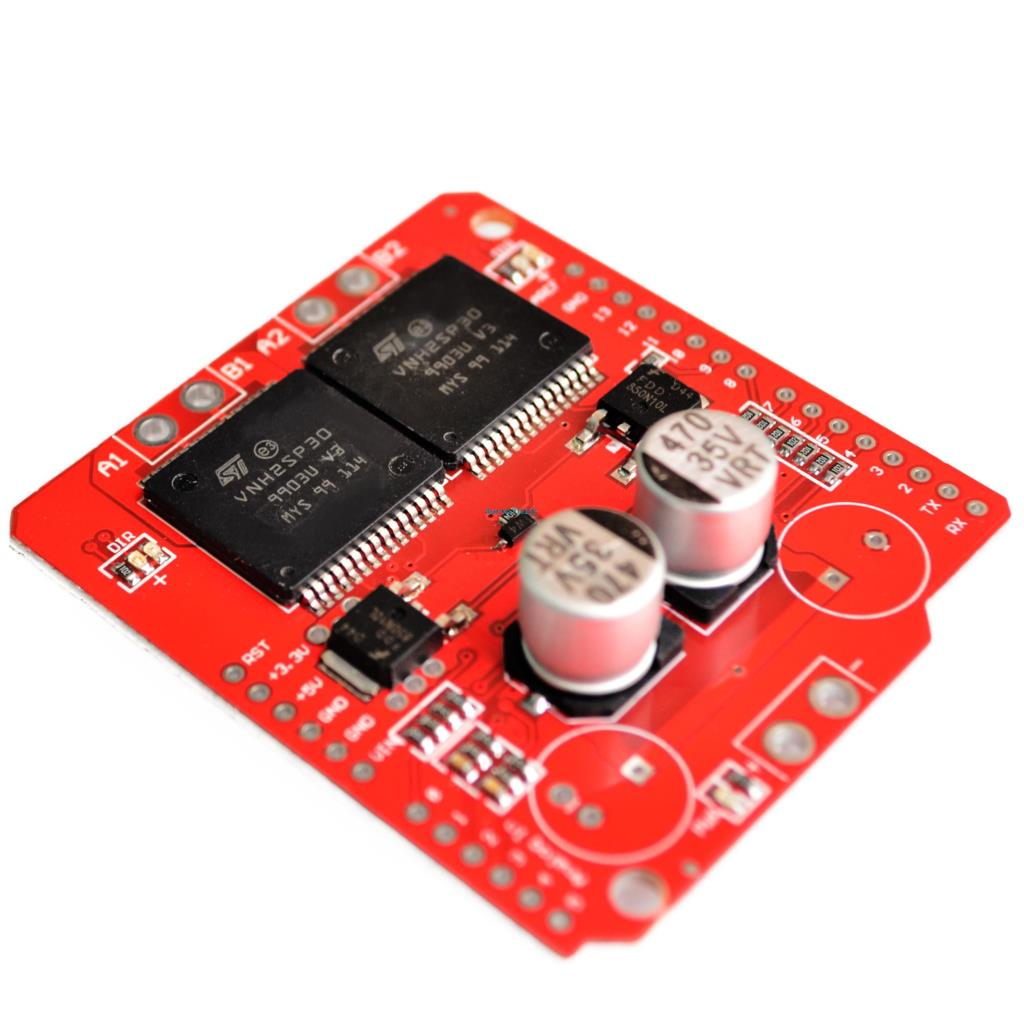https://youtu.be/gkLR8TN4kQ8?list=PLRfNwLnILuI95Y8SjcuXxxm38vTXMN0XA

AM - mode

https://youtu.be/PFGB-MBVAwk?list=PLRfNwLnILuI95Y8SjcuXxxm38vTXMN0XA

SSM - mode

`'-----------------------------------------------------------------------------------------`
`'name                     : servo DIY Driver`
`'copyright                : Bartosz Scencelek Bartcam`
`'soft                     : Ray Edgley & Bartosz Scencelek`
`'micro                    : attiny44`
`'commercial use           : no`
`'-----------------------------------------------------------------------------------------`

`\$regfile = ``"attiny44.dat"`                                   `' specify the used micro`
`\$crystal = 8000000                                          ``' used crystal frequency`

`'The following is just pinout information`
`'Port      Pin     Function Used`
`'PB0       2       Output     Motor 1 Fwd`
`'PB1       3       Output     Motor 1 Rev`
`'PB2       5       OC0A       PWM Motor 1`
`'PB3       4       Reset`
`'PA0       13      Output     Motor 2 Rev`
`'PA1       12      ADC1       Motor 1 Position`
`'PA2       11      ACD2       Motor 2 Position`
`'PA3       10      Output     Motor 2 Fwd`
`'PA4       9       SCL/Ref1   I2C Communications`
`'PA5       8       Input      Control Mode`
`'PA6       7       SDA/Ref2   I2C Communications`
`'PA7       6       OC0B       PWM Motor 2`
`'############################################`
`' Just a note on the Control Mode`
`'`
`' When set to VCC we will use the PA4 and PA6`
`' as I2C communications`
`'`
`' When set to Gnd    We will use the PA4 and PA6`
`' as PWM inputs for Ref1 and Ref2`
`'`
`' When set to 2.5V or ADC 512 we will use PA4 and PA6`
`' as Analog inputs for Ref1 and Ref2`
`'############################################`
`Dim` `Pot1 ``As` `Single`                                          `'Current Average position value Motor 1`
`Dim` `Pot2 ``As` `Single`                                          `'Current Average position value Motor 2`
`Dim` `Ref1 ``As` `Single`                                          `'Motor 1 Target Position`
`Dim` `Ref2 ``As` `Single`                                          `'Motor 2 Target Position`
`Dim` `Refcount1 ``As` `Word`
`Dim` `Refcount2 ``As` `Word`
`Dim` `Err1 ``As` `Single`
`Dim` `Err2 ``As` `Single`
`Dim` `Integral1 ``As` `Single`
`Dim` `Integral2 ``As` `Single`
`Dim` `Derivative1 ``As` `Single`
`Dim` `Derivative2 ``As` `Single`
`Dim` `Preverr1 ``As` `Single`
`Dim` `Preverr2 ``As` `Single`
`Dim` `Output1 ``As` `Single`
`Dim` `Output2 ``As` `Single`
`Dim` `Kp1 ``As` `Single`
`Dim` `Ki1 ``As` `Single`
`Dim` `Kd1 ``As` `Single`
`Dim` `Kp2 ``As` `Single`
`Dim` `Ki2 ``As` `Single`
`Dim` `Kd2 ``As` `Single`
`Dim` `Deadband1 ``As` `Single`                                     `'Motor 1 DeadBand`
`Dim` `Deadband2 ``As` `Single`                                     `'Motor 2 DeadBand`
`Dim` `Filter ``As` `Single`                                        `'Filtering Value`
`Dim` `Filter2 ``As` `Single`
`Dim` `Temp2 ``As` `Single`
`Dim` `Temp3 ``As` `Word`
`Dim` `Dt ``As` `Single`
`Dim` `Controltype ``As` `Bit`
`Dim` `Pwm_control ``As` `Byte`

`Config Portb.0 = Output                                     ``'Motor1 Fwd`
`Config Portb.1 = Output                                     ``'Motor1 Rev`
`Config Portb.2 = Output                                     ``'Motor1 PWM`
`Config Porta.0 = Output                                     ``'Motor2 Rev`
`Config Porta.3 = Output                                     ``'Motor2 Fwd`
`Config Porta.7 = Output                                     ``'Motor2 PWM`
`Config Porta.5 = Input                                      ``'input Mode "Cotrol"`
`Config Porta.2 = Input`
`Config Porta.1 = Input`

`Config Adc = ``Single` `, Prescaler = 2`
`Config Timer0 = Pwm , Compare_a_pwm = Clear_up , Compare_b_pwm = Clear_up , Prescale = 1`
`Config Timer1 = Timer , Compare_a = Disconnect , Compare_b = Disconnect , Prescale = 8`

`Controltype = Porta.5`

`'****if you want to use only one mode set controltype below****`
`' Controltype = 1`
`' Controltype = 0`

`Enable Interrupts`
`On` `Pcint0 Chint Save`
`On` `Oc1a Pwmint Save`

`If` `Controltype = 1 ``Then`                                     `'This section for PWM Input`
`   ``Enable Pcint0`
`   ``Pcmsk0 = &B01010000                                      ``'pin4 and pin6 as int`
`   ``Set` `Pcie0`
`   ``Enable Oc1a`
`   ``Start Timer1`
`   ``Tcnt1 = 0`
`   ``Pwm_control = 0`
`   ``Set` `Pcint4`
`   ``Set` `Pcint6`

`End` `If`

`Ocr0b = 0                                                   ``'Motor1 Power`
`Ocr0a = 0                                                   ``'Motor2 Power`
`' the OCR1A (Output Compare Register 1 A) is used to generate an interruput every`
`' 20 mS which is the pulse period of most PWM controlled servos.`
`' Since this controller will be working as a servo driver, we need to use`
`' the same timings.`
`' 20mS periods occure 50 times per second`
`' With a system clock of 8000000 cycles persecond, 160000 cycles will elapse each period.`
`' Timer/Counter 1 can coun up to 65535 before it over runs, so we need to use a prescaler`
`' of at least 2.441 times. This of course does not exist, so the next lowest available`
`' is 8 time. Soo if we take 8000000/8 gives us 1000000. we divide that by our 50 times`
`' per second, we get 20000, which is below the max of 65535, so we set the OCR1A to 20000`
`' if a PWM pulse exceeds the 20 mS, then we will set the ref to the current pos.`
`Ocr1a = 20000                                               ``'PWM input Timer`

`Reset Portb.0                                               ``'Motor1 Fwd`
`Reset Portb.1                                               ``'Motor1 Rev`
`Reset Porta.0                                               ``'Motor2 Rev`
`Reset Porta.3                                               ``'Motor2 Fwd`

`Start Timer0                                                ``'PWM Output Timer`

`Ref1 = 512`
`Ref2 = 512`
`Integral1 = 0`
`Integral2 = 0`
`Kp1 = 1.0                                                   ``'power force at distance`
`Ki1 = 0.4                                                   ``'power step in reaction time`
`Kd1 = 0.001                                                 ``'reaction time (higher faster)`
`Kp2 = 1.5                                                   ``'power force at distance`
`Ki2 = 0.5                                                   ``'power step in reaction time`
`Kd2 = 0.02                                                  ``'reaction time (higher faster)`
`Deadband1 = 10`
`Deadband2 = 10`
`Filter = 5                                                  ``' Set the filter to 5 Cycles`
`Filter2 = 6`
`Dt = 0.00173`
`Waitms 200`

`Do`

`'*************** Motor Feed Back with Filtering *************`
`' By taking the existing pot value and multiplying it by Filter`
`' then adding the raw pot value and deviding the total by Filter + 1`
`' We end up with a sudo average taken over filter cycles through the program`
`' The best part of this method of averaging is it is fast and doen't`
`' used much memory, the down side is it can take a long time for the average`
`' to match the raw when the raw is not moving.`
`    ``Temp3 = Getadc(1)`
`    ``Temp2 = Temp3`
`    ``Pot1 = Pot1 * Filter`
`    ``Pot1 = Pot1 + Temp2`
`    ``Pot1 = Pot1 / Filter2`

`    ``Temp3 = Getadc(2)`
`    ``Temp2 = Temp3`
`    ``Pot2 = Pot2 * Filter`
`    ``Pot2 = Pot2 + Temp2`
`    ``Pot2 = Pot2 / Filter2`

`'############################################################`
`' Control Method 2 Analog input control.`
`'############################################################`
`    ``If` `Controltype = 0 ``Then`                                 `' Analog Input Control`
`         ``Temp3 = Getadc(4)`
`         ``Temp2 = Temp3`
`         ``Ref1 = Ref1 * Filter`
`         ``Ref1 = Ref1 + Temp2`
`         ``Ref1 = Ref1 / Filter2`

`         ``Temp3 = Getadc(6)`
`         ``Temp2 = Temp3`
`         ``Ref2 = Ref2 * Filter`
`         ``Ref2 = Ref2 + Temp2`
`         ``Ref2 = Ref2 / Filter2`
`    ``Else`                                                    `' PWM input Control`
`         ``If` `Refcount1 < 19264 ``And` `Refcount1 > 17280 ``Then`
`          ``Temp3 = 19264 - Refcount1`
`          ``Temp3 = Temp3 / 1.6`

`          ``Ref1 = Ref1 * Filter`
`          ``Ref1 = Ref1 + Temp3`
`          ``Ref1 = Ref1 / Filter2`
`         ``Else`
`          ``Ref1 = Pot1`
`         ``End` `If`

`         ``If` `Refcount2 < 19264 ``And` `Refcount2 > 17280 ``Then`
`          ``Temp3 = 19264 - Refcount2`
`          ``Temp3 = Temp3 / 1.6`

`          ``Ref2 = Ref2 * Filter`
`          ``Ref2 = Ref2 + Temp3`
`          ``Ref2 = Ref2 / Filter2`
`         ``Else`
`          ``Ref2 = Pot2`
`         ``End` `If`
`    ``End` `If`

`'############################################################`
`' PID Loop to calculate the output value from the Input Error`
`'############################################################`

`    ``Err1 = Ref1 - Pot1`
`    ``Temp2 = Err1 * Dt`
`    ``Integral1 = Integral1 + Temp2`
`    ``Temp2 = Err1 - Preverr1`
`    ``Temp2 = Preverr1 + Err1`
`    ``Derivative1 = Temp2 / Dt`
`    ``Derivative1 = Derivative1 * Kd1`
`    ``Temp2 = Integral1 * Ki1`
`    ``Output1 = Kp1 * Err1`
`    ``Output1 = Output1 + Temp2`
`    ``Output1 = Output1 + Derivative1`
`    ``Preverr1 = Err1`
`    ``If` `Err1 = 0 ``Then` `Output1 = 0                            ``'end switch to GND stop`

`    ``If` `Output1 > 255 ``Then`
`         ``Output1 = 255`
`    ``Elseif Output1 < -255 ``Then`
`         ``Output1 = -255`
`    ``End` `If`

`    ``Err2 = Ref2 - Pot2`
`    ``Temp2 = Err2 * Dt`
`    ``Integral2 = Integral2 + Temp2`
`    ``Temp2 = Err2 - Preverr2`
`    ``Derivative2 = Temp2 / Dt`
`    ``Derivative2 = Derivative2 * Kd2`
`    ``Temp2 = Integral2 * Ki2`
`    ``Output2 = Kp2 * Err2`
`    ``Output2 = Output2 + Temp2`
`    ``Output2 = Output2 + Derivative2`
`    ``Preverr2 = Err2`

`    ``If` `Err2 = 0 ``Then` `Output2 = 0                            ``'end switch to GND stop`

`    ``If` `Output2 > 255 ``Then`
`         ``Output2 = 255`
`    ``Elseif Output2 < -255 ``Then`
`         ``Output2 = -255`
`    ``End` `If`

`'############################################################`
`' Drive the Motor Output and PWM signal.`
`'############################################################`
`    ``If` `Output1 > Deadband1 ``Then`                             `' Is the motor to far along`
`         ``Set` `Portb.0                                        ``' Turn on the Fwd output`
`         ``Reset Porta.0                                      ``' Turn off the Rev output`
`         ``Ocr0a = Output1                                    ``' Set the PWM to Output`
`    ``Else`
`      ``Output1 = Output1 * -1`
`      ``If` `Output1 > Deadband1 ``Then`                           `' Is the motor not far enough along`
`         ``Reset Portb.0                                      ``' Turn off the Fwd output`
`         ``Set` `Porta.0                                       ``' Turn on the Rev output`
`         ``Ocr0a = Output1                                    ``' Set the PWM to Output`
`      ``Else`
`         ``Reset Portb.0                                      ``' Turn off the Fwd output`
`         ``Reset Porta.0                                      ``' Turn off the Rev output`
`         ``Ocr0a = 0                                          ``' Turn Off the PWM`
`      ``End` `If`
`    ``End` `If`

`    ``If` `Output2 > Deadband2 ``Then`                             `' Is the motor to far along`
`         ``Set` `Porta.3                                        ``' Turn on the Fwd output`
`         ``Reset Portb.1                                      ``' Turn off the Rev output`
`         ``Ocr0b = Output2                                    ``' Set the PWM to Output`
`    ``Else`
`      ``Output2 = Output2 * -1`
`      ``If` `Output2 > Deadband2 ``Then`                           `' Is the motor not far enough along`
`         ``Reset Porta.3                                      ``' Turn off the Fwd output`
`         ``Set` `Portb.1                                        ``' Turn on the Rev output`
`         ``Ocr0b = Output2                                    ``' Set the PWM to Output`
`      ``Else`
`         ``Reset Porta.3                                      ``' Turn off the Fwd output`
`         ``Reset Portb.1                                      ``' Turn off the Rev output`
`         ``Ocr0b = 0                                          ``' Turn Off the PWM`
`      ``End` `If`
`    ``End` `If`

`Loop`

`Chint:`
`   ``If` `Pwm_control = 0 ``Then`
`      ``If` `Pina.4 = 0 ``Then`
`         ``Tcnt1 = 0`
`         ``Pwm_control = 1`
`         ``Reset Porta.4`
`      ``End` `If`
`   ``Elseif Pwm_control = 1 ``Then`
`      ``If` `Pina.4 = 1 ``Then`
`         ``Refcount1 = Tcnt1`
`         ``Tcnt1 = 0`
`         ``Set` `Porta.6`
`         ``Pcmsk0 = &B01000000`
`         ``Pwm_control = 2`
`      ``End` `If`
`   ``Elseif Pwm_control = 2 ``Then`
`      ``If` `Pina.6 = 0 ``Then`
`         ``Tcnt1 = 0`
`         ``Pwm_control = 3`
`         ``Reset Porta.6`
`      ``End` `If`
`   ``Elseif Pwm_control = 3 ``Then`
`      ``If` `Pina.6 = 1 ``Then`
`         ``Refcount2 = Tcnt1`
`         ``Tcnt1 = 0`
`         ``Set` `Porta.4`
`         ``Pcmsk0 = &B00010000`
`         ``Pwm_control = 0`
`      ``End` `If`
`   ``End` `If`

`Return`

`Pwmint:`
`   ``If` `Pwm_control = 0 ``Or` `Pwm_control = 1 ``Then`
`      ``Pwm_control = 2`
`      ``Pcmsk0 = &B01000000`
`      ``Refcount1 = 0`
`   ``Elseif Pwm_control = 2 ``Or` `Pwm_control = 3 ``Then`
`      ``Pwm_control = 0`
`      ``Pcmsk0 = &B00010000`
`      ``Refcount2 = 0`
`   ``End` `If`
`Tcnt1 = 0`
`Return`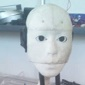### solutions of sterring

first option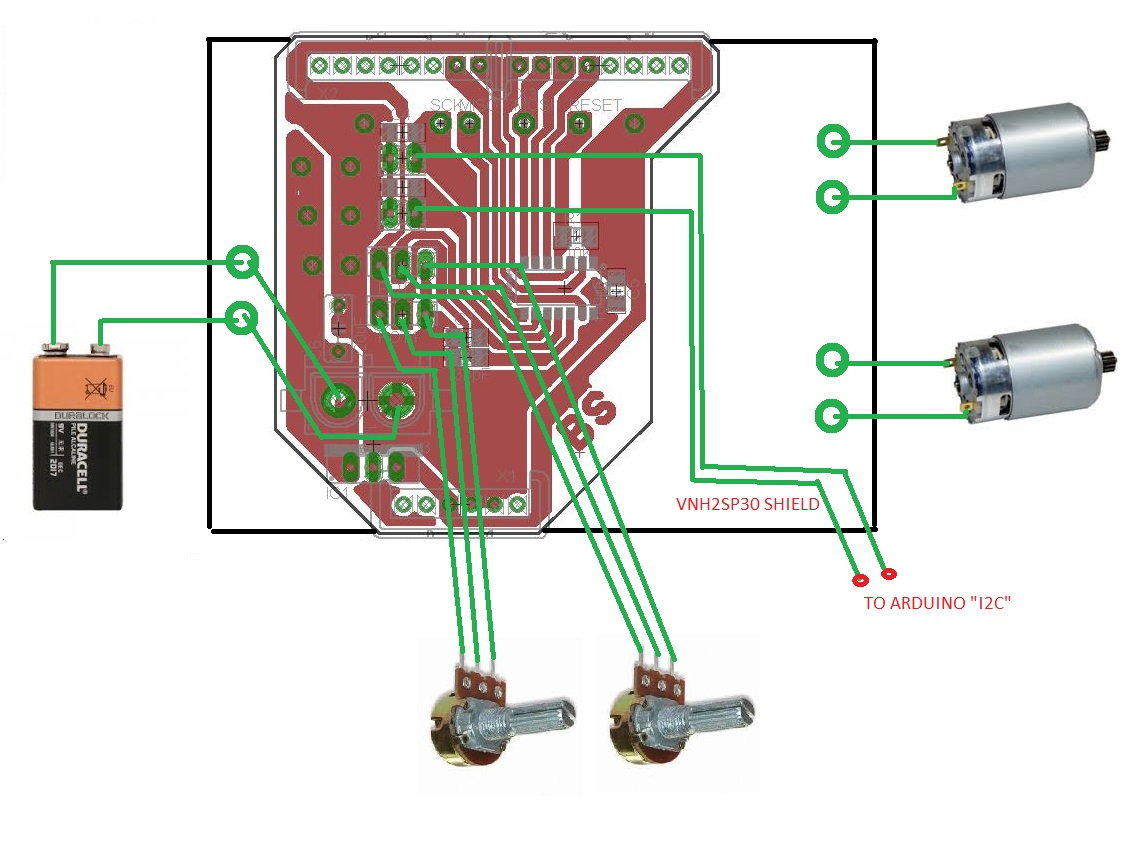second option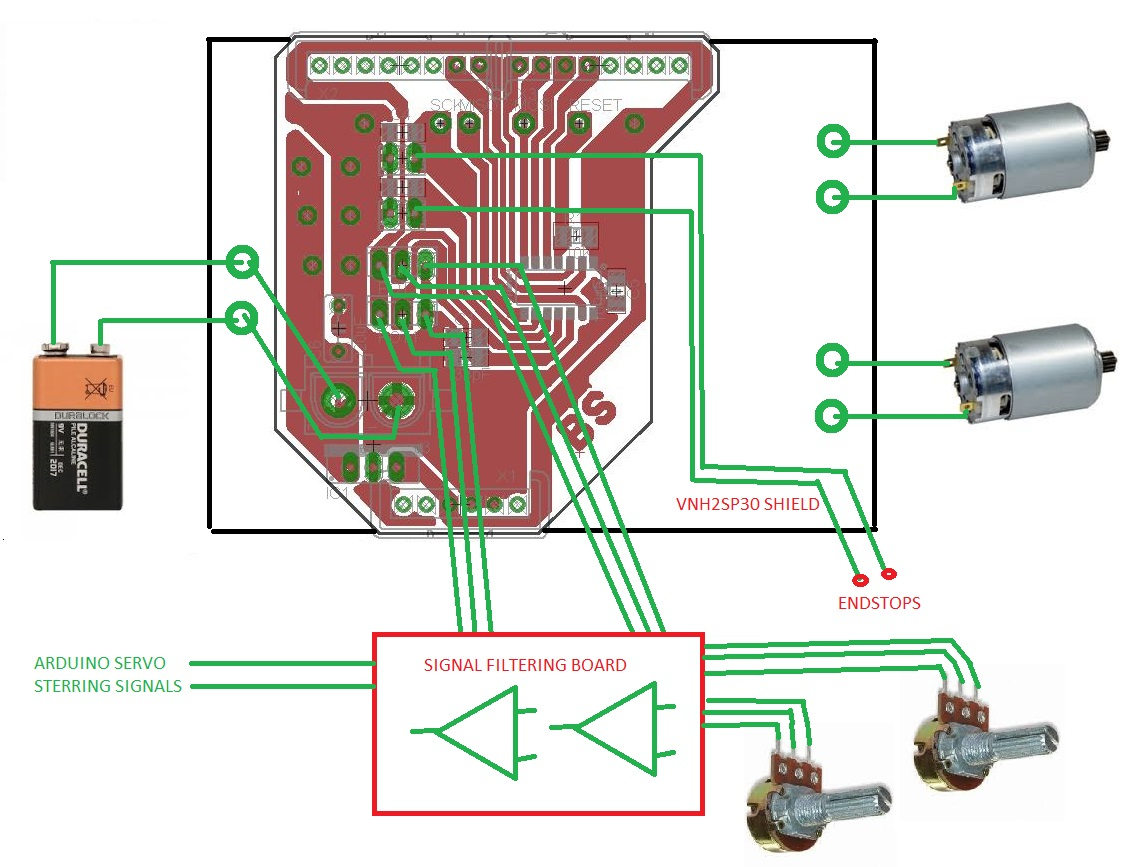and this is what i have now, only for testing manualy all mechanism (soft is for this and need to write it to other solutions) , most importand is the dynamics of motor stering. Manually I check all solutions of motors dynamics. Motors must be fast but with correct acceleration and braking (manually may check all of the solutions)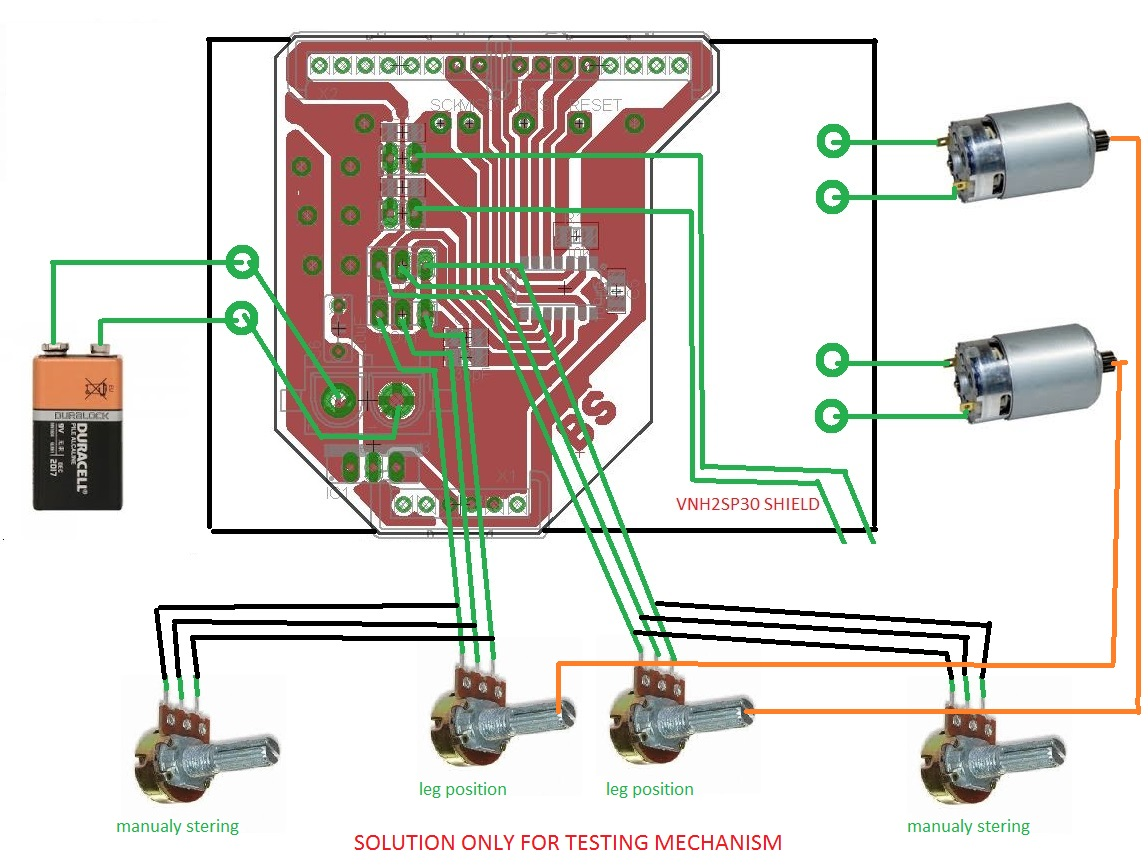### Bartcam as Discussed

This is the start of the proposed pin usage

'Port      Pin     Function Used
'PB0       2       Motor 1 Fwd
'PB1       3       Motor 1 Rev
'PB2       5       OC0A    PWM Motor 1
'PB3       4       Motor 2 Fwd
'PA0       13     Motor 2 Rev
'PA1       12      ADC1    Motor 1 Position
'PA2       11      ACD2    Motor 2 Position
'PA3       10
'PA4       9       SCL     I2C Communications
'PA5       8
'PA6       7       SDA     I2C Communications
'PA7       6       OC0B    PWM Motor 2### Try this out Bartcam

Hello Bart,

Try out the following code.

it uses over 80% of the Flash memory but does have PID control with a choice of Analog control or PWM input like an RC servo.  Output is dual cahnnel PWM.

'-----------------------------------------------------------------------------------------
'name                     : servo DIY Driver
'micro                    : attiny44
'commercial use           : no
'-----------------------------------------------------------------------------------------

\$regfile = "attiny44.dat"                                   ' specify the used micro
\$crystal = 8000000                                          ' used crystal frequency

'The following is just pinout information
'Port      Pin     Function Used
'PB0       2       Output     Motor 1 Fwd
'PB1       3       Output     Motor 1 Rev
'PB2       5       OC0A       PWM Motor 1
'PB3       4       Reset
'PA0       13      Output     Motor 2 Rev
'PA1       12      ADC1       Motor 1 Position
'PA2       11      ACD2       Motor 2 Position
'PA3       10      Output     Motor 2 Fwd
'PA4       9       SCL/Ref1   I2C Communications
'PA5       8       Input      Control Mode
'PA6       7       SDA/Ref2   I2C Communications
'PA7       6       OC0B       PWM Motor 2
'############################################
' Just a note on the Control Mode
'
' When set to VCC we will use the PA4 and PA6
' as I2C communications
'
' When set to Gnd    We will use the PA4 and PA6
' as PWM inputs for Ref1 and Ref2
'
' When set to 2.5V or ADC 512 we will use PA4 and PA6
'############################################
Dim Pot1 As Single                                          'Current Average position value Motor 1
Dim Pot2 As Single                                          'Current Average position value Motor 2
Dim Ref1 As Single                                          'Motor 1 Target Position
Dim Ref2 As Single                                          'Motor 2 Target Position
Dim Refcount1 As Word
Dim Refcount2 As Word
Dim Err1 As Single
Dim Err2 As Single
Dim Integral1 As Single
Dim Integral2 As Single
Dim Derivative1 As Single
Dim Derivative2 As Single
Dim Preverr1 As Single
Dim Preverr2 As Single
Dim Output1 As Single
Dim Output2 As Single
Dim Kp1 As Single
Dim Ki1 As Single
Dim Kd1 As Single
Dim Kp2 As Single
Dim Ki2 As Single
Dim Kd2 As Single
Dim Filter As Single                                        'Filtering Value
Dim Filter2 As Single
'Dim Temp As String * 10
Dim Temp2 As Single
Dim Temp3 As Word
Dim Dt As Single
Dim Controltype As Bit
Dim Pwm_control As Byte

On Pcint0 Chint Save
On Oc1a Pwmint Save

Config Portb.0 = Output                                     'Motor1 Fwd
Config Portb.1 = Output                                     'Motor1 Rev
Config Portb.2 = Output                                     'Motor1 PWM
Config Porta.0 = Output                                     'Motor2 Rev
Config Porta.3 = Output                                     'Motor2 Fwd
Config Porta.7 = Output                                     'Motor2 PWM
Config Porta.5 = Input                                      'input Mode "Cotrol"

Config Adc = Single , Prescaler = 2
Config Timer0 = Pwm , Compare_a_pwm = Clear_up , Compare_b_pwm = Clear_up , Prescale = 8
Config Timer1 = Timer , Compare_a = Disconnect , Compare_b = Disconnect , Prescale = 8

Enable Interrupts

Ocr0b = 0                                                   'Motor1 Power
Ocr0a = 0                                                   'Motor2 Power
' the OCR1A (Output Compare Register 1 A) is used to generate an interruput every
' 20 mS which is the pulse period of most PWM controlled servos.
' Since this controller will be working as a servo driver, we need to use
' the same timings.
' 20mS periods occure 50 times per second
' With a system clock of 8000000 cycles persecond, 160000 cycles will elapse each period.
' Timer/Counter 1 can coun up to 65535 before it over runs, so we need to use a prescaler
' of at least 2.441 times. This of course does not exist, so the next lowest available
' is 8 time. Soo if we take 8000000/8 gives us 1000000. we divide that by our 50 times
' per second, we get 20000, which is below the max of 65535, so we set the OCR1A to 20000
' if a PWM pulse exceeds the 20 mS, then we will set the ref to the current pos.
Ocr1a = 20000                                               'PWM input Timer

Reset Portb.0                                               'Motor1 Fwd
Reset Portb.1                                               'Motor1 Rev
Reset Porta.0                                               'Motor2 Rev
Reset Porta.3                                               'Motor2 Fwd
Controltype = Porta.5

Start Timer0                                                'PWM Output Timer

If Controltype = 1 Then                                     'This section for PWM Input
Set Pcie0
Enable Oc1a
Start Timer1
Tcnt1 = 0
Pwm_control = 0
Set Pcint4
End If

Ref1 = 512
Ref2 = 512
Integral1 = 0
Integral2 = 0
Kp1 = 0.1
Ki1 = 0.1
Kd1 = 0
Filter = 5                                                  ' Set the filter to 5 Cycles
Filter2 = Filter + 1
Dt = 0.00173
Waitms 200

Do

'*************** Motor Feed Back with Filtering *************
' By taking the existing pot value and multiplying it by Filter
' then adding the raw pot value and deviding the total by Filter + 1
' We end up with a sudo average taken over filter cycles through the program
' The best part of this method of averaging is it is fast and doen't
' used much memory, the down side is it can take a long time for the average
' to match the raw when the raw is not moving.
Temp2 = Temp3
Pot1 = Pot1 * Filter
Pot1 = Pot1 + Temp2
Pot1 = Pot1 / Filter2

Temp2 = Temp3
Pot2 = Pot2 * Filter
Pot2 = Pot2 + Temp2
Pot2 = Pot2 / Filter2

'############################################################
'############################################################
Temp2 = Temp3
Ref1 = Ref1 * Filter
Ref1 = Ref1 + Temp2
Ref1 = Ref1 / Filter2

Temp2 = Temp3
Ref2 = Ref2 * Filter
Ref2 = Ref2 + Temp2
Ref2 = Ref2 / Filter2
Else                                                    ' PWM input Control
If Refcount1 < 1000 Or Refcount1 > 2000 Then       ' if the Refcount is not in range
Ref1 = Pot1                                     'set the Ref to be equal to the current position
Else
Temp3 = Refcount1 - 988
Ref1 = Temp3
End If
If Refcount2 < 1000 Or Refcount2 > 2000 Then
Ref2 = Pot2
Else
Temp3 = Refcount2 - 988
Ref2 = Temp3
End If
End If

'############################################################
' PID Loop to calculate the output value from the Input Error
'############################################################

Err1 = Ref1 - Pot1
Temp2 = Err1 * Dt
Integral1 = Integral1 + Temp2
Temp2 = Err1 - Preverr1
Derivative1 = Temp2 / Dt
Derivative1 = Derivative1 * Kd1
Temp2 = Integral1 * Ki1
Output1 = Kp1 * Err1
Output1 = Output1 + Temp2
Output1 = Output1 + Derivative1
Preverr1 = Err1
If Output1 > 255 Then
Output1 = 255
Elseif Output1 < -255 Then
Output1 = -255
End If

Err2 = Ref2 - Pot2
Temp2 = Err2 * Dt
Integral2 = Integral2 + Temp2
Temp2 = Err2 - Preverr2
Derivative2 = Temp2 / Dt
Derivative2 = Derivative2 * Kd2
Temp2 = Integral2 * Ki2
Output2 = Kp2 * Err2
Output2 = Output2 + Temp2
Output2 = Output2 + Derivative2
Preverr2 = Err2

'############################################################
' Drive the Motor Output and PWM signal.
'############################################################
If Output1 > Deadband1 Then                             ' Is the motor to far along
Set Portb.0                                        ' Turn on the Fwd output
Reset Portb.1                                      ' Turn off the Rev output
Ocr0a = Output1                                    ' Set the PWM to Output
Else
Output1 = Output1 * -1
If Output1 > Deadband1 Then                           ' Is the motor not far enough along
Reset Portb.0                                      ' Turn off the Fwd output
Set Portb.1                                        ' Turn on the Rev output
Ocr0a = Output1                                    ' Set the PWM to Output
Else
Reset Portb.0                                      ' Turn off the Fwd output
Reset Portb.1                                      ' Turn off the Rev output
Ocr0a = 0                                          ' Turn Off the PWM
End If
End If

If Output2 > Deadband2 Then                             ' Is the motor to far along
Set Porta.3                                        ' Turn on the Fwd output
Reset Porta.0                                      ' Turn off the Rev output
Ocr0b = Output2                                    ' Set the PWM to Output
Else
Output2 = Output2 * -1
If Output2 > Deadband2 Then                           ' Is the motor not far enough along
Reset Porta.3                                      ' Turn off the Fwd output
Set Porta.0                                        ' Turn on the Rev output
Ocr0b = Output2                                    ' Set the PWM to Output
Else
Reset Porta.3                                      ' Turn off the Fwd output
Reset Porta.0                                      ' Turn off the Rev output
Ocr0b = 0                                          ' Turn Off the PWM
End If
End If

Loop

Chint:
If Pwm_control = 0 Then
If Porta.4 = 0 Then
Tcnt1 = 0
Pwm_control = 1
End If
Elseif Pwm_control = 1 Then
If Porta.4 = 1 Then
Refcount1 = Tcnt1
Tcnt1 = 0
Reset Pcmsk0.4
Set Pcint6
Pwm_control = 2
End If
Elseif Pwm_control = 2 Then
If Porta.6 = 0 Then
Tcnt1 = 0
Pwm_control = 3
End If
Elseif Pwm_control = 3 Then
If Porta.4 = 1 Then
Refcount2 = Tcnt1
Tcnt1 = 0
Reset Pcmsk0.6
Set Pcint4
Pwm_control = 0
End If
End If
Return

Pwmint:
If Pwm_control = 0 Or Pwm_control = 1 Then
Reset Pcmsk0.4
Set Pcint6
Pwm_control = 2
Refcount1 = 0
Tcnt1 = 0
Elseif Pwm_control = 2 Or Pwm_control = 3 Then
Reset Pcmsk0.6
Set Pcint4
Pwm_control = 0
Refcount2 = 0
Tcnt1 = 0
End If
Return
------------------------------------------------------------------------------------------------------------------------------------
Please let me know how it goes.

It seams the I2C library is only available in the comercial paid version of Bascom and I don't think it's worth it.

Ray### OK, i modify PCB and check

OK, i modify PCB and check this out. I read all the code and it seems to be Very OK. I am impressed Ray!### Do let me know how it

Do let me know how it goes.

There are ome serious limitations with this chip, and the programming software.

But it will be interesting to know if it works.

Ray### Chip may be changed to other

Chip may be changed to other like atmega or xmega..### Wow Bartcam ! Such a great

Wow Bartcam !
Such a great post with your excellent mobile platform solutions.
Quality work - thanks for posting.### PB3 - is the reset pin of

Thank You Grog, and thank You Ray...

PB3 - is the reset pin of microcontroller (if I swich off this i can not program it)

ok i try to project new PCB for those, I must think about how to comunicate by I2C without any breaks in ADC, on int, we must do some sub funcion where we get signals from I2C.

We have one pin free, this may be used for some endstop switch, better will be to use two pins , one for each motor..### problem with filtering

There is problem with filtering in program, if I use

output1=ref1-pot1

without this filtering:

Err1 = Ref1 - Pot1
Temp2 = Err1 * Dt
Integral1 = Integral1 + Temp2
Temp2 = Err1 - Preverr1
Derivative1 = Temp2 / Dt
Derivative1 = Derivative1 * Kd1
Temp2 = Integral1 * Ki1
Output1 = Kp1 * Err1
Output1 = Output1 + Temp2
Output1 = Output1 + Derivative1
Preverr1 = Err1

with this filtering all works in only one direction, I belive there is some small mistake in calculations. I try to find this ;/

all it's working in analog mode, I don't checked PWM and I2C mode yet.

Sorry no I2C mode ... yet!

Ok, i find one problem:

Derivative1 = Derivative1 * Kd1

Derivative1 will be always =0 because Kd1 is =0

hmmm filter is working in excel maybe is something with variables in bascom### errors in simulation

that I am think about in  simulations in bascom of filtering is many errors.
I cutted the code and do a small of it to simulation only:

\$regfile = "attiny44.dat"                                   ' specify the used micro
\$crystal = 8000000                                          ' used crystal frequency

'The following is just pinout information
'Port      Pin     Function Used
'PB0       2       Output     Motor 1 Fwd
'PB1       3       Output     Motor 1 Rev
'PB2       5       OC0A       PWM Motor 1
'PB3       4       Reset
'PA0       13      Output     Motor 2 Rev
'PA1       12      ADC1       Motor 1 Position
'PA2       11      ACD2       Motor 2 Position
'PA3       10      Output     Motor 2 Fwd
'PA4       9       SCL/Ref1   I2C Communications
'PA5       8       Input      Control Mode
'PA6       7       SDA/Ref2   I2C Communications
'PA7       6       OC0B       PWM Motor 2
'############################################
' Just a note on the Control Mode
'
' When set to VCC we will use the PA4 and PA6
' as I2C communications
'
' When set to Gnd    We will use the PA4 and PA6
' as PWM inputs for Ref1 and Ref2
'
' When set to 2.5V or ADC 512 we will use PA4 and PA6
'############################################
Dim Pot1 As Single                                          'Current Average position value Motor 1
Dim Pot2 As Single                                          'Current Average position value Motor 2
Dim Ref1 As Single                                          'Motor 1 Target Position
Dim Ref2 As Single                                          'Motor 2 Target Position
Dim Refcount1 As Word
Dim Refcount2 As Word
Dim Err1 As Single
Dim Err2 As Single
Dim Integral1 As Single
Dim Integral2 As Single
Dim Derivative1 As Single
Dim Derivative2 As Single
Dim Preverr1 As Single
Dim Preverr2 As Single
Dim Output1 As Single
Dim Output2 As Single
Dim Kp1 As Single
Dim Ki1 As Single
Dim Kd1 As Single
Dim Kp2 As Single
Dim Ki2 As Single
Dim Kd2 As Single
Dim Filter As Single                                        'Filtering Value
Dim Filter2 As Single
'Dim Temp As String * 10
Dim Temp2 As Single
Dim Temp3 As Word
Dim Dt As Single
Dim X As Single

Ref1 = 512
Ref2 = 512
Integral1 = 0
Integral2 = 0
Kp1 = 0.1
Ki1 = 0.1
Kd1 = 0.1
Filter = 5                                                  ' Set the filter to 5 Cycles
Filter2 = 6
Dt = 0.00173
Pot1 = 510
Ref1 = 512

Do

Err1 = Ref1 - Pot1
Temp2 = Err1 * Dt
Integral1 = Integral1 + Temp2
Temp2 = Err1 - Preverr1
Derivative1 = Temp2 / Dt
Derivative1 = Derivative1 * Kd1
Temp2 = Integral1 * Ki1
Output1 = Kp1 * Err1
Output1 = Output1 + Temp2
Output1 = Output1 + Derivative1
Preverr1 = Err1
X = Output1

'

Loop

The X is calculation of what is end value to output. I don't know where is erroe in calculations, must check each by each### Is it the interrupts?

Is it possible the error is comming from an external source such as the two timer interrupts?

There are quite a number of interrupts on the tiny84, i used one for the two pwm outputs, and one is usec for the pwm input timing!### At last work on analog mode

At last work on analog mode with PID :)

but, no live with PWM mode, I connected normal servo to check signal from the arduino and normal servo its working , an these same pin DIY SERV DRV not work. I thin there is something with INT.

.

.

.

.

OK i found there is no ENABLE PCINT(X)

SET PCINT(x) - what for ? I think it is not neccecery.

Tommorow is next day of checking :P### Possible correction

Hello Bartca,

I noticed that you are running with higher counts than was origonally calculated, not sure where I got it wrong,

In one of the comments you made you mentioned having to restric the range due to instability, There is a setting that may not have been taken into account.

Up the the setup section, we set OCR1A to 20000, with the real world results we are seeing this may be should have been closer to 40000.

Just a thought

The OCR1A is used to trigger the interrupt for loss of signal in what you called SSM mode (I called it PWM mode, but your naming will lead to less confusion so I will match your naming)

Ray### Who was able to run this

Who was able to run this driver? SSM mode does not work for me